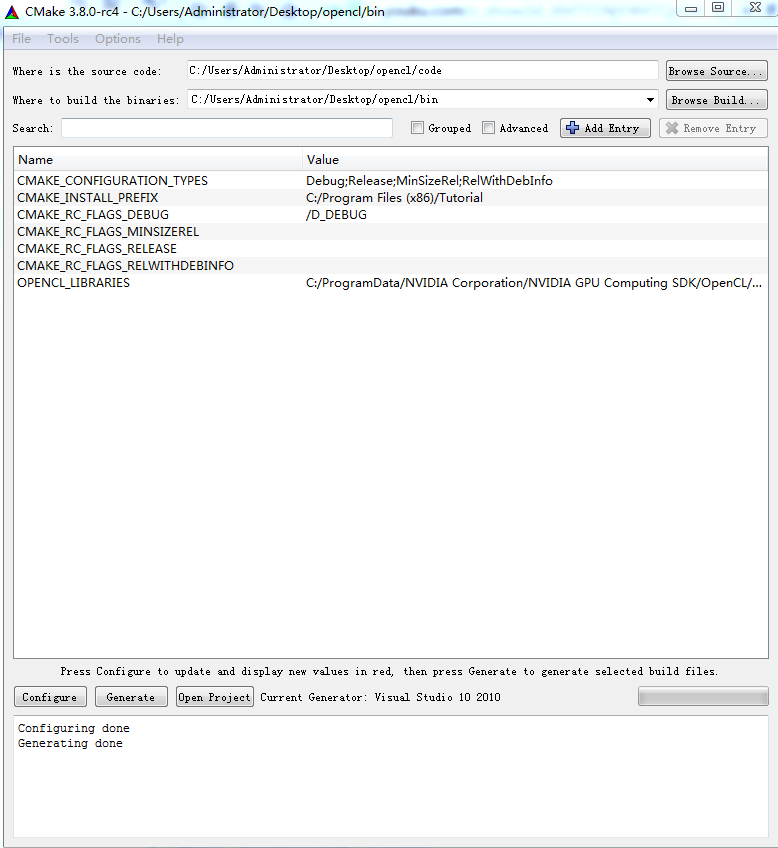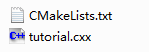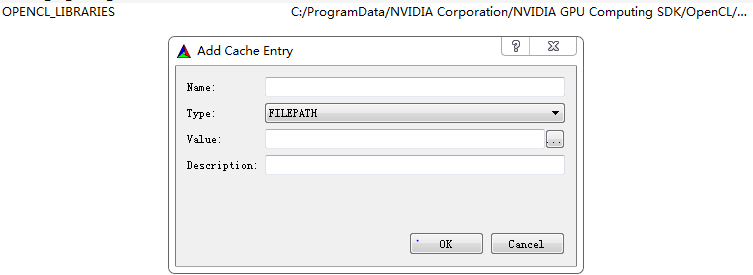# OPENCL菜鸟学习——环境配置【windows+vs】

1.CMake

2.NVIDIA GPU Computing SDK

3.visual studio 2010cmake_minimum_required (VERSION 2.6)
project (Tutorial)
add_executable(Tutorial tutorial.cxx)

tutorial.cxx中为需要编译的代码

#include <stdio.h>
#include <stdlib.h>
#include <math.h>
int main (int argc, char *argv[])
{
if (argc < 2)
{
fprintf(stdout,"Usage: %s number\n",argv);
return 1;
}
double inputValue = atof(argv);
double outputValue = sqrt(inputValue);
fprintf(stdout,"The square root of %g is %g\n",
inputValue, outputValue);
return 0;
}

/bin中会生成对应的app等vs编译中间件。vs中会出现